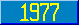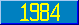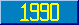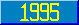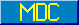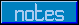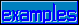## expr

### expr

Introduced in the 1977 ANSI M[UMPS] language standard.

This metalanguage symbol represents an expression. An expression (formula) starts with a basic value denoting object (expratom). Optionally, there may be more. If there is, first comes a (binary) operator, and then another basic value denoting object. The binary operator, in some cases, may be negated by a not sign ('). This additional part is called an exprtail. An expression may have any number of exprtails.

### exprtail

Introduced in the 1977 ANSI M[UMPS] language standard.

This metalanguage symbol represents the tail end of an expression. (See the metalanguage symbol expr).

### logicalop

Introduced in the 1977 ANSI M[UMPS] language standard.

This metalanguage symbol represents any of the logical binary operators, i.e. one of ! or &.

Approved for addition in a future M[UMPS] language standard.

The logical operator !! (exclusive or) is added to the list of possible operators.

### truthop

Introduced in the 1977 ANSI M[UMPS] language standard.

This metalanguage symbol represents any of the relational binary operators (i.e. one of =, <, >, [ or ]) or any of the logical binary operators, (i.e. one of !, or &).